# Python | forget_pack() and forget_grid() method in Tkinter

• Last Updated : 29 Nov, 2021

If we want to unmap any widget from the screen or toplevel then` forget()` method is used. There are two types of forget method `forget_pack()` ( similar to `forget() `) and `forget_grid()` which are used with `pack()` and `grid()` method respectively.

### `forget_pack()` method –

```Syntax: widget.forget_pack()

widget can be any valid widget which is visible.
```
Code #1:

 `# Imports tkinter and ttk module``from` `tkinter ``import` `*` `from` `tkinter.ttk ``import` `*`` ` `# toplevel window``root ``=` `Tk()`` ` `# method to make widget invisible``# or remove from toplevel``def` `forget(widget):`` ` `    ``# This will remove the widget from toplevel``    ``# basically widget do not get deleted``    ``# it just becomes invisible and loses its position``    ``# and can be retrieve``    ``widget.forget()`` ` `# method to make widget visible``def` `retrieve(widget):``    ``widget.pack(fill ``=` `BOTH, expand ``=` `True``)`` ` `# Button widgets``b1 ``=` `Button(root, text ``=` `"Btn 1"``)``b1.pack(fill ``=` `BOTH, expand ``=` `True``)`` ` `# See, in command forget() method is passed``b2 ``=` `Button(root, text ``=` `"Btn 2"``, command ``=` `lambda` `: forget(b1))``b2.pack(fill ``=` `BOTH, expand ``=` `True``)`` ` `# In command retrieve() method is passed``b3 ``=` `Button(root, text ``=` `"Btn 3"``, command ``=` `lambda` `: retrieve(b1))``b3.pack(fill ``=` `BOTH, expand ``=` `True``)`` ` `# infinite loop, interrupted by keyboard or mouse``mainloop()`

Output: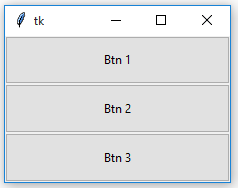After forget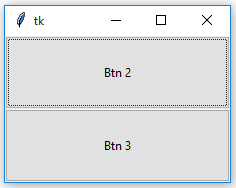After retrieval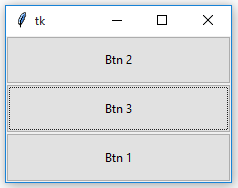Notice the difference in the position of Button 1 before and after forget as well as after retrieval.

### `forget_grid()` method –

```Syntax: widget.forget_grid()

widget can be any valid widget which is visible.
```

Note : This method can be used only with `grid() `geometry methods.

Code #2:

 `# Imports tkinter and ttk module``from` `tkinter ``import` `*``from` `tkinter.ttk ``import` `*`` ` `# toplevel window``root ``=` `Tk()`` ` `# method to make widget invisible``# or remove from toplevel``def` `forget(widget):`` ` `    ``# This will remove the widget from toplevel``    ``# basically widget do not get deleted``    ``# it just becomes invisible and loses its position``    ``# and can be retrieve``    ``widget.grid_forget()`` ` `# method to make widget visible``def` `retrieve(widget):``    ``widget.grid(row ``=` `0``, column ``=` `0``, ipady ``=` `10``, pady ``=` `10``, padx ``=` `5``)`` ` `# Button widgets``b1 ``=` `Button(root, text ``=` `"Btn 1"``)``b1.grid(row ``=` `0``, column ``=` `0``, ipady ``=` `10``, pady ``=` `10``, padx ``=` `5``)`` ` `# See, in command forget() method is passed``b2 ``=` `Button(root, text ``=` `"Btn 2"``, command ``=` `lambda` `: forget(b1))``b2.grid(row ``=` `0``, column ``=` `1``, ipady ``=` `10``, pady ``=` `10``, padx ``=` `5``)`` ` `# In command retrieve() method is passed``b3 ``=` `Button(root, text ``=` `"Btn 3"``, command ``=` `lambda` `: retrieve(b1))``b3.grid(row ``=` `0``, column ``=` `2``, ipady ``=` `10``, pady ``=` `10``, padx ``=` `5``)`` ` `# infinite loop, interrupted by keyboard or mouse``mainloop()`

Output: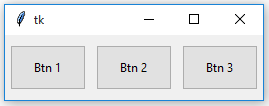After Forget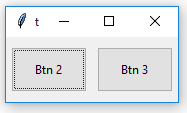After Retrieval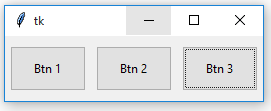Notice that the position of Button 1 remains same after forget and retrieval. With `grid_forget()` method, you can place it at any grid after retrieval but generally, the original grid is chosen.

My Personal Notes arrow_drop_up